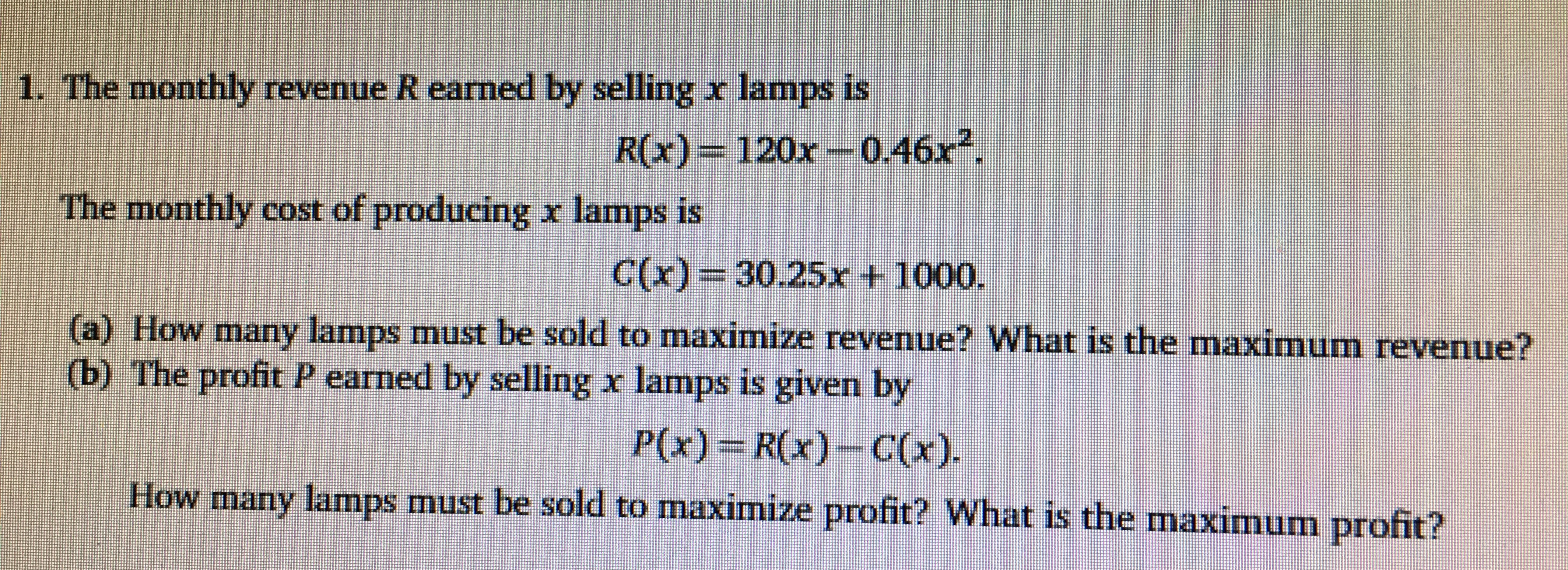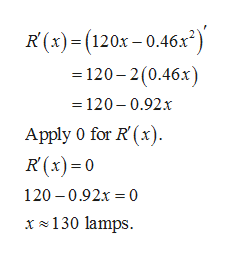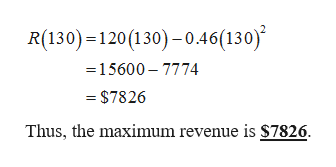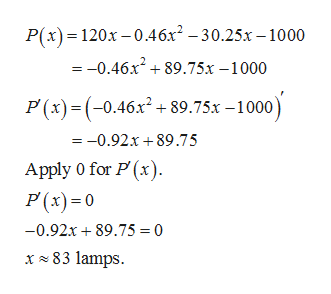1. The monthly revenue R earned by selling x lamps isR(x)= 120x-0.46xThe monthly cost of producing x lamps isC(x) 30.25r t 1000.a) How marny lamps must be sold to maximize revenue? What is the maximum revenue?(b) The profit P earned by selling x lamps is given byP(x) R(x)-C(x)How many lamnps must be sold to maximize profit? What is the maximum profit?

Questionhelp_outlineImage Transcriptionclose1. The monthly revenue R earned by selling x lamps is R(x)= 120x-0.46x The monthly cost of producing x lamps is C(x) 30.25r t 1000. a) How marny lamps must be sold to maximize revenue? What is the maximum revenue? (b) The profit P earned by selling x lamps is given by P(x) R(x)-C(x) How many lamnps must be sold to maximize profit? What is the maximum profit? fullscreen
Step 1

(a) The given revenue and cost functions are,help_outlineImage TranscriptioncloseR(x)(120x-0.46x) = 120-2(0.46x) 120-0.92.x Apply 0 for R (x) R(x)0 120 0.92x 0 x130 lamps fullscreen
Step 2

Approximately 130 lamps must to be sold for the maximum revenue.

Substitute 130 for x in revenue function to find the maximum revenue.help_outlineImage TranscriptioncloseR(130) 120(130)-0.46(130) =15600 7774 - \$7826 Thus, the maximum revenue is \$7826 fullscreen
Step 3

(b)Find maximum...help_outlineImage TranscriptioncloseP(x) 120x-0.46x2 -30.25x -1000 =-0.46x89.75x-1000 P(x)(0.46x+89.75x -1000) = -0.92x 89.75 Apply 0 for P (x) P (x)0 -0.92x 89.75 =0 x83 lamps fullscreen

Want to see the full answer?

See Solution

Want to see this answer and more?

Our solutions are written by experts, many with advanced degrees, and available 24/7

See Solution
Tagged in

Other